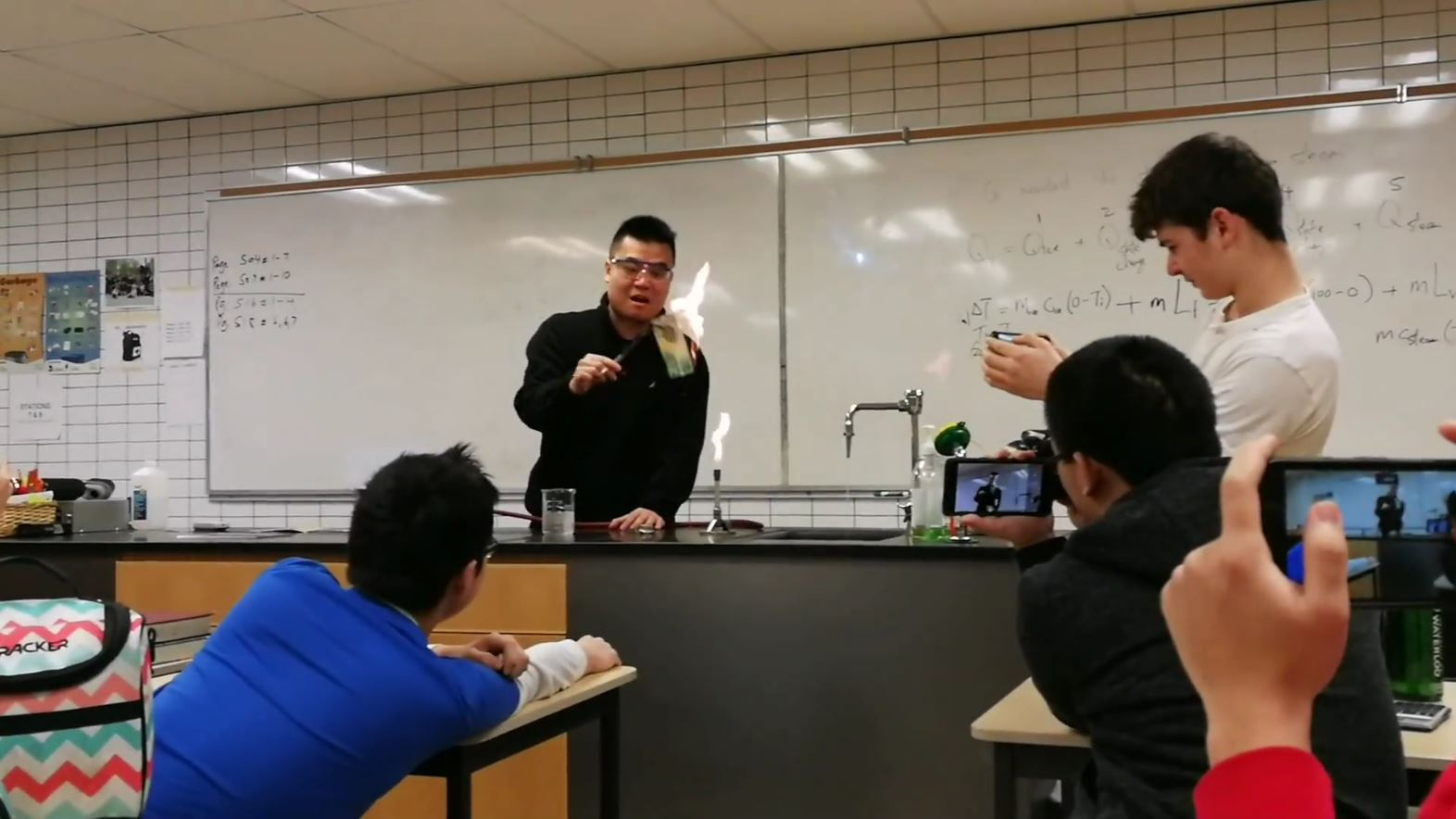## Solving Heat Problems

In the past few days, we explored some thermal energy problems!

In particular, we examined two substances reaching thermal equilibrium: simple and complex calorimetry problems! Here’s a summary:

1) Two substances in contact with each other will eventually reach thermal equilibrium. For this to happen, heat must transfer from the hotter substance to the colder substance. Provided that the system is closed and energy is conserved, we know that the energy gained by the colder substance is equal to the energy lost by the warmer substance.

2) With simple calorimetry, the solution to final temperature of the two substances is fairly straightforward. None of the substances change state, so we can just use specific heat.

Energy Gained by Substance A (colder) = Energy Lost by Substance B (warmer)

QA = -QB

mAcAΔTA = -mBcBΔTB

mAcA(T2 - T1-A) = -mBcB(T2 - T1-B)

We can try this out with this sample problem:

A 200 g block of copper at 80.0 ℃ is placed in a tank of 800 g of water at 20.0 ℃. What will be the final temperature of the water?

The specific heat of copper is 385 J kg-1 K-1 and the specific heat of water is 4187 J kg-1 K-1.

mwatercwater(T2 - T1-water) = -mcopperccopper(T- T1-copper)

0.800(4187)(T2 - 20.0) = -0.200(385)(T2 - 80.0)

0.800(4187)T2 - 20.0(0.800)(4187) = -0.200(385)T2 + 80.0(0.200)(385)

0.800(4187)T2 + 0.200(385)T2 = 20.0(0.800)(4187) + 80.0(0.200)(385)

(0.800(4187) + 0.200(385))T2 = 66992 + 6160

(3349.6 + 77)T2 = 73152

(3426.6)T2 = 73152

T2 ≅ 21.3 ℃

The final temperature of the water is about 21.3 ℃.

3) With complex calorimetry, we need to consider all forms of thermal energy transferred. This includes the latent heat from a change of state, as well as different specific heat capacity of the different states of a substance.

Energy Gained by Substance A (colder) = Energy Lost by Substance B (warmer)

∑QA = -∑QB

We can try this out with this sample problem:

A 37 g ice cube at -15 ℃ is dropped into cup of 2.0 kg of hot water at 50 ℃. What will be the final temperature of the water?

The specific heat of ice is 2108 J kg-1 K-1, the specific heat of water is 4187 J kg-1 K-1, and the latent heat of fusion is 334 000 J kg-1.

Let the ice be substance A and the water be substance B

QA(s) + QA(f) + QA(l) = -QB

mAcA(s)(0 - (-15)) + mALfA + mAcA(l)(T2 - 0) = -mBcB(T2 - T1-B)

(0.037)(2108)(15) + (0.037)(334000) + (0.037)(4187)(T2) = -(2.0)(4187)(T2 - 50)

13527.94 + 0.037(4187)T2 = -2.0(4187)T2 + 50(2.0)(4187)

(0.037(4187) + 2.0(4187))T2 = 418700 - 13527.94

(154.919 + 8374)T2 = 405172.06

T2 ≅ 48 ℃

The final temperature of the water is about 48 ℃.

Two questions I still have about heat and the math behind it:

1) What affects the rate of transfer of heat? So far, we’ve only found what affects how much heat is transferred.

2) Experimentally, will we get the same values of specific heat and latent heat of the theoretical values? If not, why? What affects the specific heat and latent heat of a substance?

An intriguing experiment we did today with the quiz involved burning money, without actually burning it! The way it works is that the fire does not actually come from the combustion of the paper, instead it comes from the combustion of the alcohol of the alcohol-water solution the paper was dipped in. Since the solution also has water, the high specific heat capacity of the water is able to absorb the heat and protect the paper from increasing in temperature and combusting!

#### After Unit Reflection

##### Solving Energy Transformation Problems

In this blog post, I solved heat transfer problems involving the transformation of thermal energy in one body to thermal energy in another body. I worked on simple calorimetry problems with two bodies reaching thermal equilibrium. To solve these problems, I used the law of conservation of energy to equate Qa = -Qb and the equation for the heat capacity of a body: Q = mcΔT. In addition to simple calorimetry problems, I worked on complex calorimetry problems that involved heat capacity and latent heat. I had to use the equation for the latent heat of a body, Q = mL, and used the law of conservation of energy in its transformation into increasing the internal kinetic energy of the other body.